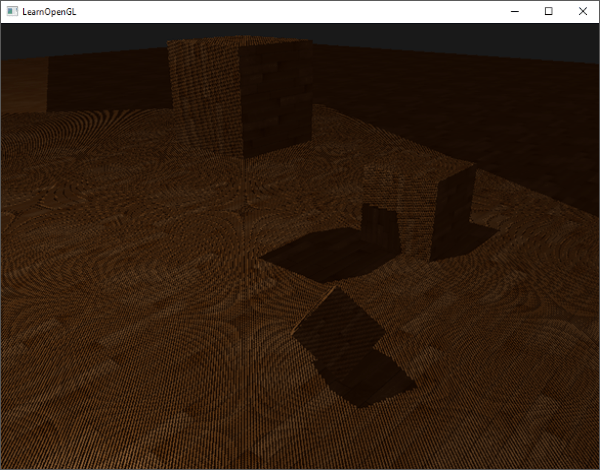$$f(z) = H(d-z) = \begin{cases} 0,&d<z\\ 1,&d\geq z \end{cases}$$

SSM存在以下几个主要问题：$$bias = units * r + factor * dz$$

## 二. Percentage Closer Filtering(PCF)

PCF的基本原理是在像素周围区域内多次采样并进行深度值比较，然后对结果进行加权平均，即

$$f(z) = \sum_i H(d_i-z_i)w_i$$

### 2.1 硬件PCF

DX上则更简单，DX9直接使用tex2Dproj函数即可，DX11则使用SampleCmp函数。‘

### 2.2 软件PCF### 2.3 PCF的优劣

#### 优点

• 实现简单
• 硬件直接提供支持
• 很容易与PCSS等动态半影算法相结合

#### 缺点

• 边缘有噪点
• 采样数增多时，开销较大

VSM由Donnelly和Lauritzen于2006年提出，它用一种基于统计的方法来计算shadow。核心原理是，将shadow map看成深度的分布$$F$$，Donnelly和Lauritzen证明了当shadow receiver为平面时，shadow的值等于$$F$$的切比雪夫不等式的上界

$$f(z) = \frac{\sigma^2}{\sigma^2+(z-d)^2}$$

$$\sigma^2 = E(d^2)-E^2(d)$$

### 3.1 光渗现象（Light Bleeding)$$E(z) = \frac{a+b}{2} \\ E(z^2) = \frac{a^2+b^2}{2}$$

$$d = \frac{a+b}{2} \\ \sigma^2 = \frac{(a-b)^2}{4}$$

$$f(z) = \frac{\sigma^2}{\sigma^2+(c-d)^2} = \frac{(\frac{\Delta x}{\Delta y})^2} {2(\frac{\Delta x}{\Delta y})^2 + 4\frac{\Delta x}{\Delta y} + 4}$$

### 3.3 其他注意事项

$$f(z) = e^{-cz}* F$$

Lauritzen注意到，shadow计算的本质是比较$$z$$和$$d$$。但事实上我们可以不用直接比较$$z$$和$$d$$的值，而是套一个warp function $$g$$，比较$$g(z)$$和$$g(d)$$，也能得到一样的结果。在此情况下，仍然可以通过计算切比雪夫不等式上限来得到阴影的值。

warp function可以任选，而EVSM选取的warp function正是ESM中采用的$$e^{cd}$$。因此，EVSM的步骤和VSM非常类似，区别在于现在shadow map中存放$$e^{cd}$$和$$e^{2cd}$$而不是$$d$$和$$d^2$$，以及在计算切比雪夫不等式上限时使用$$e^{cz}$$而不是$$z$$。

EVSM仍然可以采用VSM中提到的remapping技术来消除光渗，这样它就有了双重手段，有两个参数可以调节：$$c$$和最小阴影值$$m$$。通过适当调节参数可以得到非常高质量的阴影。

## 参考文献

Williams, Lance. "Casting curved shadows on curved surfaces." ACM Siggraph Computer Graphics. Vol. 12. No. 3. ACM, 1978.

Reeves, William T., David H. Salesin, and Robert L. Cook. "Rendering antialiased shadows with depth maps." ACM Siggraph Computer Graphics. Vol. 21. No. 4. ACM, 1987.

Uralsky, Yury. "Efficient soft-edged shadows using pixel shader branching." GPU Gems 2 (2005): 269-282.

Donnelly, William, and Andrew Lauritzen. "Variance shadow maps." Proceedings of the 2006 symposium on Interactive 3D graphics and games. ACM, 2006.

Lauritzen, Andrew Timothy. Rendering antialiased shadows using warped variance shadow maps. MS thesis. University of Waterloo, 2008.

Lauritzen, Andrew, and Michael Mccool. "Summed-area variance shadow maps." GPU Gems. 2007.

Annen, Thomas, et al. "Exponential shadow maps." Graphics Interface. ACM Press, 2008.

Wright, Daniel. "Dynamic occlusion with signed distance fields." ACM SIGGRAPH. 2015.### 留下你的评论

*评论支持代码高亮<pre class="prettyprint linenums">代码</pre>

### 相关推荐

#### PBR理论体系整理（三）：IBL